# Toy cars

Pavel has a collection of toy cars. He wanted to regroup them. But in the division of three, four, six, and eight, he was always one left. Only when he formed groups of seven, he divided everyone. How many toy cars have in the collection?

Correct result:

n =  49

#### Solution: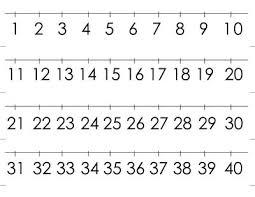We would be pleased if you find an error in the word problem, spelling mistakes, or inaccuracies and send it to us. Thank you!## Next similar math problems:

• Test pointsIf you earned 80% of the possible 40 points, how many points did you miss to get 100%?
• RemainderA is an arbitrary integer that gives remainder 1 in the division with 6. B is an arbitrary integer that gives remainder 2 the division by. What makes remainder in division by 3 product of numbers A x B ?
• Divisible by 5How many three-digit odd numbers divisible by 5, which are in place ten's number 3?
• Walnutsx walnuts were in the mission. Dano took 1/4 of nuts Michael took 1/8 from the rest and John took 34 nuts. It stayed here 29 nuts. Determine the original number of nuts.
• In the orchardIn the orchard, they planted 25 apple trees, 20 pears, 15 plums and 40 marbles. A strong late frost, however, destroyed a fifth of all new trees. Unfortunately, it was all the trees of one kind of fruit. What is the probability that the plums have died ou
• Mom and daughterMother is 39 years old. Her daughter is 15 years. For many years will mother be four times older than the daughter?
• Three-digitHow many three-digit natural numbers do not have the number 7?
• Three daysDuring the three days sold in stationery 1490 workbooks. The first day sold about workbooks more than third day. The second day 190 workbooks sold less than third day. How many workbooks sold during each day?
• Three friendsThe three friends spent 600 KC in a teahouse. Thomas paid twice as much as Paul. Paul a half less than Zdeněk. How many each paid?
• Aircraft modelsHow many car models did the collector own after the collector's market? The collector of car and aircraft models owned 3 times more car models than aircraft. He sold 7 car models and 9 aircraft models on the stock exchange and bought 12 car models and 4 a
• Air draftThe numbers 1,2,3,4,5 are written on five tickets on the table. Air draft randomly shuffled the tickets and composed a 5-digit number from them. What is the probability that he passed: and, the largest possible number b, the smallest possible number c, a
• Cows and calves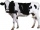There are 168 cows and calves in the cowshed. Cows are in nine stalls and calves in four stalls. Same count cows are in every cow stall and three more in each calf stall than in a cow stall. What is the capacity of the stalls for cows and what for calves?
• Tunnels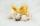Mice had built an underground house consisting of chambers and tunnels: • each tunnel leading from the chamber to the chamber (none is blind) • from each chamber lead just three tunnels into three distinct chambers, • from each chamber mice can get to any
• Four-digit numbers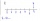Find four-digit numbers where all the digits are different. For numbers, the sum of the third and fourth digits is twice the sum of the first two digits, and the sum of the first and fourth digits is equal to the sum of the second and third digits. The diIn six baskets, the seller has fruit. In individual baskets, there are only apples or just pears with the following number of fruits: 5,6,12,14,23 and 29. "If I sell this basket," the salesman thinks, "then I will have just as many apples as a pear." Whic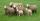Shepherd tending the sheep. Tourists asked him how much they have. The shepherd said, "there are fewer than 500. If I them lined up in 4-row 3 remain. If in 5-row 4 remain. If in 6-row 5 remain. But I can form 7-row." How many sheep have herdsman?Paľo, Jano, Karol, and Rišo were doing an intelligence test. Palo correctly answered half of the questions plus 7 questions, Jano to a third plus 18 questions, Karol to a quarter plus 21 questions and Risho to a fifth plus 25 questions. After the test, Ka